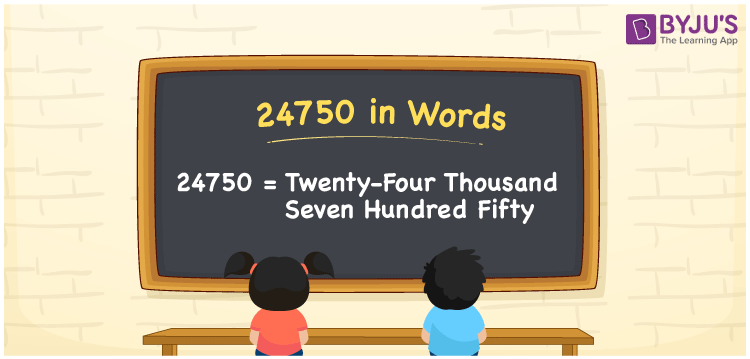# 24750 in Words

24750 in words is Twenty-four Thousand Seven Hundred Fifty. For example, if you have 24750 rupees in your account, then you can write, “I have Twenty-four Thousand Seven Hundred Fifty rupees in my account”. We know that the number name of any number can be written using the ones, tens, hundreds and thousands place of a number. Therefore, the place value chart is essential to write 24750 in words. 24750 is a cardinal number as it shows a specific quantity.

 24750 in words Twenty-four Thousand Seven Hundred Fifty Twenty-four Thousand Seven Hundred Fifty in Numbers 24750

## 24750 in English Words## How to Write 24750 in Words?

We can convert 24750 to words using a place value chart. This can be done as follows. The number 24750 has 5 digits, so let’s make a chart that shows the place value up to 5 digits.

 Ten thousands Thousands Hundreds Tens Ones 2 4 7 5 0

Thus, we can write the expanded form as:

2 × Ten thousand + 4 × Thousand + 7 × Hundred + 5 × Ten + 0 × One

= 2 × 10000 + 4 × 1000 + 7 × 100 + 5 × 10 + 0 × 1

= 24750

= Twenty-four Thousand Seven Hundred Fifty

24750 is the natural number that is succeeded by 24749 and preceded by 24751.

24750 in words – Twenty-four Thousand Seven Hundred Fifty

Is 24750 an odd number? – No.

Is 24750 an even number? – Yes

Is 24750 a perfect square number? – No

Is 24750 a perfect cube number? – No

Is 24750 a prime number? – No

Is 24750 a composite number? – Yes

## Frequently Asked Questions on 24750 in Words

Q1

### How to write 24750 in words?

24750 in English is written as “Twenty-four Thousand Seven Hundred Fifty”.
Q2

### Is the number 24750 an odd number?

No, the number 24750 is not an odd number.
Q3

### Is 24750 a composite number?

Yes, 24750 is a composite number.